# GMAT Math : DSQ: Calculating percents

## Example Questions

← Previous 1

### Example Question #1 : Percents

What percent of students in a school are freshmen with glasses?

(1) Of the freshmen in the school, 10% wear glasses.

(2) Of the non-freshmen in the school, 20% wear glasses.

BOTH statements (1) and (2) TOGETHER are sufficient to answer the question asked, but NEITHER statement ALONE is sufficient to answer the question asked.

Statement (1) ALONE is sufficient, but statement (2) alone is not sufficient to answer the question asked.

Statement (2) ALONE is sufficient, but statement (1) alone is not sufficient to answer the question asked.

Statements (1) and (2) TOGETHER are NOT sufficient to answer the question asked, and additional data specific to the problem are needed.

Statements (1) and (2) TOGETHER are NOT sufficient to answer the question asked, and additional data specific to the problem are needed.

Explanation:

To answer this question, it is necessary to know the total number of students in the school and the number of freshmen with glasses.

(1) This indicates that 10% of the freshmen have glasses; neither the total number of freshmen nor the total number of students in the school is provided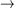NOT sufficient.

(2) Provides the percent of non-freshmen who wear glasses (irrelevant to the question at hand). It does not provide the total number of students in the school or the number of freshmen with glassesNOT sufficient.

From (1) and (2),  the percent of non-freshmen with glasses is known and the percent of the freshmen with glasses is known, but not the percent of the school who are freshmen with glasses.

For example:

If there are 100 freshmen, including 10 freshmen with glasses, and 100 non-freshmen, including 20 non-freshmen with glasses, then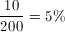of the school are freshmen with glasses.

If there are 300 freshmen, including 30 freshmen with glasses and 100 non-freshmen, including 20 non-freshmen with glasses, then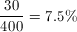of the school are freshmen with glasses.

Both statements together are not sufficient.

### Example Question #2 : Percents

In 2013 there are 300 employees at Company ABC. If the number of employees at Company ABC increased by 200% from 1993 to 2013, by what percent did the number of employees at Company ABC increase from 2003 to 2013?

(1) In 2003 there were 160 employees at Company ABC.

(2) From 1993 to 2003 the number of employees increased by 60% at Company ABC.

E. Statements (1) and (2) TOGETHER are NOT sufficient.

B. Statement (2) ALONE is sufficient, but statement (1) alone is not sufficient.

A. Statement (1) ALONE is sufficient, but statement (2) alone is not sufficient.

C. BOTH statements TOGETHER are sufficient, but NEITHER statement ALONE is sufficient.

D. EACH statement ALONE is sufficient.

D. EACH statement ALONE is sufficient.

Explanation:

For statement (1), since we know the numbers of employees in 2003 and 2013, we can directly calculate the percentage change: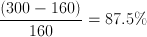.

For statement (2), since we know the percentage change from 1993 to 2013 and the percentage change from 1993 to 2003, we can set the percentage change from 2003 to 2013 to beand then calculatefrom the following: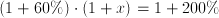.

Solveand we can get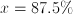.

### Example Question #3 : Percents

Data sufficiency question- do not actually solve the question

How many male students are in a class?

1. There are 42 students in the class.

2. 55 percent of the students are female.

Both statements taken together are sufficienct to answer the question, but neither statement alone is sufficient

Statement 2 alone is sufficient, but statement 1 along is not sufficient to answer the question

Statement 1 alone is sufficient but statement 2 alone is not sufficient to answer the question

Each statement alone is sufficient

Statements 1 and 2 together are not sufficient, and additional data is neeeded to answer the question

Both statements taken together are sufficienct to answer the question, but neither statement alone is sufficient

Explanation:

In order to calculate the number of males in the class, you need to know the total number of students and the number of females (which can be calculated using the percentage).

### Example Question #4 : Percents

Data sufficiency question- do not actually solve the question

There are 20 cats in an animal shelter. How many black, female cats are in the shelter?

1. 14 of the cats are male

2. 25 percent of the cats are black

Statement 1 alone is sufficient, but statement 2 is not sufficient to answer the question

Statement 2 alone is sufficient, but statement 1 is not sufficient to answer the question

Both statements taken together are sufficient to answer the question, but neither statement alone is sufficient

Statements 1 and 2 together are not sufficient, and additional data is needed to answer the question

Each statement alone is sufficient to answer the question

Statements 1 and 2 together are not sufficient, and additional data is needed to answer the question

Explanation:

The information provided will allow you to calculate the number of females and the number of black cats, but there is not enough information to quantify the number of black, female cats

### Example Question #5 : Percents

A certain pet store sells cats and dogs. The number of dogs is 250% greater than the number of cats. How many cats are in the store?

1. There are 40 dogs in the store.

2. There are 56 cats and dogs in the store.

Statement (1) and (2) TOGETHER are NOT sufficient to answer the question asked, and additional data are needed.

BOTH statements TOGETHER are sufficient, but NEITHER statement ALONE is sufficient.

Statement (1) ALONE is sufficient, but statement (2) alone is not sufficient.

Statement (2) ALONE is sufficient, but statement (1) alone is not sufficient.

EACH statement ALONE is sufficient.

EACH statement ALONE is sufficient.

Explanation: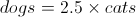Statement 1: sufficient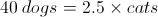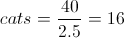Statement 2: sufficient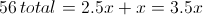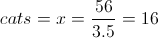### Example Question #6 : Percents

Mrs. Smith purchased groceries whose price, before tax, was $147.64. What was the tax rate on those groceries (nearest hundredth of a percent)? 1) The tax she paid was$9.23.

2) The total amount she paid was $156.87 Possible Answers: Statement 1 ALONE is sufficient to answer the question, but Statement 2 ALONE is not sufficient. EITHER Statement 1 or Statement 2 ALONE is sufficient to answer the question. BOTH statements TOGETHER are sufficient to answer the question, but NEITHER statement ALONE is sufficient to answer the question. Statement 2 ALONE is sufficient to answer the question, but Statement 1 ALONE is not sufficient. BOTH statements TOGETHER are NOT sufficient to answer the question. Correct answer: EITHER Statement 1 or Statement 2 ALONE is sufficient to answer the question. Explanation: To determine the tax rate, you need to know the purchase price, which is given, and the amount of tax paid. The amount of tax is given in Statement 1. Statement 2 alone, however, also allows you to find the amount of tax; just subtract: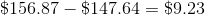Either way, you can now find the tax rate: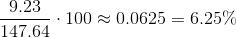### Example Question #7 : Percents Jared, a jewelry salesman, makes a 10% commission on the selling price of all the jewelry he sells. Last month, he earned$4,000 in commission. What is the total selling price of the jewelry he sold this month?

1. His sales this month were 25% more than his sales last month.

2. He earned $5,000 in commission this month. Possible Answers: EACH statement ALONE is sufficient to answer the question asked. Statement (1) ALONE is sufficient, but statement (2) alone is not sufficient to answer the question asked. Statements (1) and (2) TOGETHER are NOT sufficient to answer the question asked, and additional data specific to the problem are needed. Statement (2) ALONE is sufficient, but statement (1) alone is not sufficient to answer the question asked. BOTH statements (1) and (2) TOGETHER are sufficient to answer the question asked, but NEITHER statement ALONE is sufficient to answer the question asked. Correct answer: EACH statement ALONE is sufficient to answer the question asked. Explanation: Each statement has enough information to calculate the answer. Using statement 1, we know that he sold 25% more than last month. Given in the question, last month he earned$4,000. If $4,000 is 10% of the total price of his jewelry sold last month, we do some math (below) to find he sold$40,000 worth of jewelry. Letbe the total price of jewelry sold last month. Then,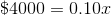so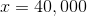Increasing that by 25% we see that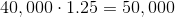NOTE: when multiplying the percents, make sure you use the decimal values.

Using statement 2, we know that we can just calculate the total selling price of the jewelry he sold this month based on the commission percentage. So, if we letequal the total price of jewelry sold this month, we get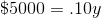or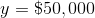Therefore we see we can use either statement individually to find what we are looking for.

### Example Question #8 : Percents

A distributor ordered 3,250 chairs.  2,315 chairs were delivered.  What percentage of the chairs have not yet been delivered?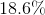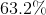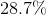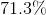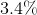Explanation:

First let's find the number of chairs not delivered: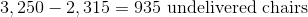Therefore the fraction of chairs not delivered is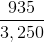.

Divide the numerator by the denominator to convert this fraction into a decimal: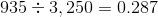Multiply by 100 to turn this decimal into a percentage: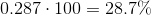### Example Question #9 : Percents

What percent of a company's employees are women with a college degree?

(1) Of the women employed in the company,do not have a college degree.

(2) Of the men employed in the company,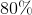have a college degree.

Both statements TOGETHER are not sufficient.

Each statement ALONE is sufficient.

Statement (1) ALONE is sufficient, but Statement (2) ALONE is not sufficient

Statement (2) ALONE is sufficient, but Statement (1) ALONE is not sufficient

Both statements TOGETHER are sufficient, but NEITHER statement ALONE is sufficient

Both statements TOGETHER are not sufficient.

Explanation:

Statement (1) indicates the percentage of women who do not have a college degree. From that statement, we know that 60% of the women in the company have a college degree. However, we do not know the total number of employees or the total number of women in the company. Therefore, statement (1) alone is not sufficient.

Statement (2) indicates the percentage of men who have a college degree but from that statement we cannot find the total number of employees or the total number of women with a college degree.

We need the total number of women and the total number of employees in order to calculate the percentage of employees who are women with a college degree. We show it with the following example:

If we assume that there are 100 women and 100 men working in the company, we can attempt to find the percentage of employees who are women with a college degree in the following way: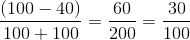So in that example, 30% of the employees are women with a college degree.

However if we change the number of women to 500 and the number of men to 600, the percentage of employees who are women with a college degree is: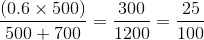The percentage of employees who are women with a college degree becomes 25%.

Therefore both statements together are not sufficient.

### Example Question #10 : Percentsis 30% of, and 60% of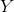andare positive integers.

True or false:is a positive integer.

Statement 1:is a multiple of 5.

Statement 2:is a multiple of 5.

BOTH statements TOGETHER are insufficient to answer the question.

BOTH statements TOGETHER are sufficient to answer the question, but NEITHER statement ALONE is sufficient to answer the question.

Statement 2 ALONE is sufficient to answer the question, but Statement 1 ALONE is NOT sufficient to answer the question.

EITHER statement ALONE is sufficient to answer the question.

Statement 1 ALONE is sufficient to answer the question, but Statement 2 ALONE is NOT sufficient to answer the question.

Statement 2 ALONE is sufficient to answer the question, but Statement 1 ALONE is NOT sufficient to answer the question.

Explanation:

Statement 1 alone is inconclusive. For example, if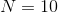, thenis 30% of 10 - that is,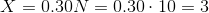,

an integer.

But if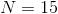, thenis 30% of 15 - that is,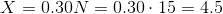,

not an integer.

If Statement 2 alone is assumed, then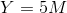for some integer. 60% of this is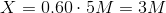.is three times an integer and is itself an integer.

← Previous 1

Tired of practice problems?

Try live online GMAT prep today.